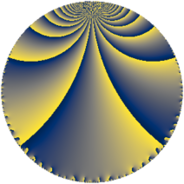# Properties

 Label 46.4.cLevel $46$ Weight $4$ Character orbit 46.c Rep. character $\chi_{46}(3,\cdot)$ Character field $\Q(\zeta_{11})$ Dimension $60$ Newform subspaces $2$ Sturm bound $24$ Trace bound $2$

# Related objects

## Defining parameters

 Level: $$N$$ $$=$$ $$46 = 2 \cdot 23$$ Weight: $$k$$ $$=$$ $$4$$ Character orbit: $$[\chi]$$ $$=$$ 46.c (of order $$11$$ and degree $$10$$) Character conductor: $$\operatorname{cond}(\chi)$$ $$=$$ $$23$$ Character field: $$\Q(\zeta_{11})$$ Newform subspaces: $$2$$ Sturm bound: $$24$$ Trace bound: $$2$$ Distinguishing $$T_p$$: $$3$$

## Dimensions

The following table gives the dimensions of various subspaces of $$M_{4}(46, [\chi])$$.

Total New Old
Modular forms 200 60 140
Cusp forms 160 60 100
Eisenstein series 40 0 40

## Trace form

 $$60 q + 8 q^{3} - 24 q^{4} + 10 q^{5} + 8 q^{6} - 8 q^{7} - 146 q^{9} + O(q^{10})$$ $$60 q + 8 q^{3} - 24 q^{4} + 10 q^{5} + 8 q^{6} - 8 q^{7} - 146 q^{9} + 20 q^{10} + 34 q^{11} + 32 q^{12} + 112 q^{13} - 40 q^{14} + 744 q^{15} - 96 q^{16} + 148 q^{17} + 40 q^{18} - 258 q^{19} - 312 q^{20} - 1060 q^{21} - 296 q^{22} - 968 q^{23} + 32 q^{24} - 782 q^{25} - 412 q^{26} + 272 q^{27} + 144 q^{28} + 716 q^{29} + 1016 q^{30} + 1256 q^{31} + 988 q^{33} + 520 q^{34} + 886 q^{35} - 584 q^{36} + 894 q^{37} + 436 q^{38} + 312 q^{39} + 80 q^{40} - 1034 q^{41} + 64 q^{42} - 1350 q^{43} + 136 q^{44} - 2644 q^{45} - 92 q^{46} - 3256 q^{47} + 128 q^{48} - 1024 q^{49} - 728 q^{50} + 328 q^{51} + 448 q^{52} - 448 q^{53} + 3252 q^{54} + 5564 q^{55} + 1776 q^{56} + 9364 q^{57} + 1552 q^{58} + 3814 q^{59} + 72 q^{60} - 414 q^{61} - 868 q^{62} - 4600 q^{63} - 384 q^{64} - 6368 q^{65} - 5360 q^{66} - 3330 q^{67} - 3104 q^{68} - 7756 q^{69} - 5968 q^{70} - 5512 q^{71} - 1952 q^{72} - 1132 q^{73} - 3564 q^{74} + 4410 q^{75} - 152 q^{76} + 224 q^{77} + 812 q^{78} + 3178 q^{79} + 1568 q^{80} + 4126 q^{81} + 4000 q^{82} + 6808 q^{83} + 4824 q^{84} + 6262 q^{85} + 3856 q^{86} - 2090 q^{87} + 752 q^{88} - 826 q^{89} + 4804 q^{90} - 2048 q^{91} - 3804 q^{93} - 1008 q^{94} - 272 q^{95} + 128 q^{96} + 11758 q^{97} + 6120 q^{98} + 8964 q^{99} + O(q^{100})$$

## Decomposition of $$S_{4}^{\mathrm{new}}(46, [\chi])$$ into newform subspaces

Label Dim $A$ Field CM Traces $q$-expansion
$a_{2}$ $a_{3}$ $a_{5}$ $a_{7}$
46.4.c.a $30$ $2.714$ None $$-6$$ $$6$$ $$10$$ $$107$$
46.4.c.b $30$ $2.714$ None $$6$$ $$2$$ $$0$$ $$-115$$

## Decomposition of $$S_{4}^{\mathrm{old}}(46, [\chi])$$ into lower level spaces

$$S_{4}^{\mathrm{old}}(46, [\chi]) \cong$$ $$S_{4}^{\mathrm{new}}(23, [\chi])$$$$^{\oplus 2}$$